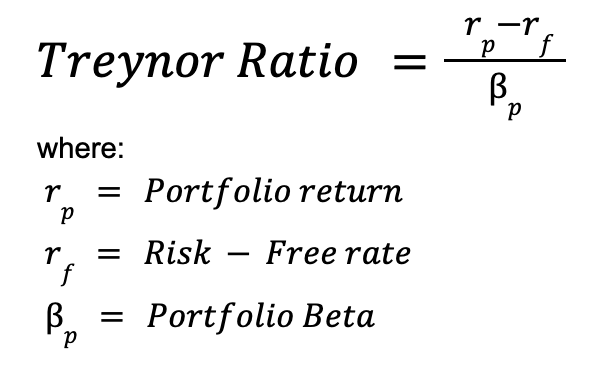Start tracking your investments with Portseido today
Try Portseido for free, and explore all the tools you need to track and plan all your investments.
Home Portseido Blog Treynor Ratio - What is it? How to calculate?

# Treynor Ratio - What is it? How to calculate?

Article last updated: September 28, 2023## What is the Treynor Ratio?

The Treynor ratio, also known as Treynor Index, reward-to-volatility ratio or Treynor measure, is the financial metric that measures portfolio performance adjusted by systematic risk. It helps investors to assess how much excess return generated for additional risk taken.

## The Treynor Ratio FormulaIn the Treynor ratio calculation, there are two main components:

### 1. Excess return

This refers to portfolio return in excess to risk-free rate. This is represented by the numerator of the equation which is the portfolio return minus by risk-free rate. Portfolio return is the portfolio actual return over the given period of time. While the risk-free rate is the rate of the return of a risk-free asset which is usually assumed to be the treasury bond of the same currency.

### 2. Beta

Beta (β) is a financial metric measuring the volatility of the asset or portfolio return compared to its benchmark.

## Is a higher or lower Treynor ratio better?

The higher the Treynor Ratio is generally considered better. A higher ratio signifies that the investment or portfolio is generating more return per unit of systematic risk (as measured by beta). This suggests that the investment is providing a better risk-adjusted return.

## Treynor ratio vs Sharpe ratio

The Treynor ratio and Sharpe ratio have many characteristics in common since they both measure risk-adjusted return for portfolio. The only difference between the two is how they measure risk related to the investment. While Treynor ratio uses Beta which is a portfolio return volatility relative to market’s (systematic risk), Sharpe ratio uses the actual portfolio return volatility (total risk).

## Limitations of the Treynor Ratio

Although Treynor ratio is a good metric in measuring risk-adjusted return, there is no one perfect metric. Here are a few limitations of Treynor Ratio:

### 1. Negative Beta

The Treynor Ratio loses its meaning if Beta is negative. Therefore, to use the Treynor Ratio effectively, Beta must be positive.

### 2. Beta as a Risk Measure

Beta may not always be a perfect measurement of risk. It quantifies an underlying investment's volatility relative to its benchmark, which might not fully capture its risk profile. Relying solely on Beta may overlook certain nuances of risk associated with the investment.

### 3. Treynor Ratio as an Ordinal Number

The Treynor Ratio is an ordinal number, meaning it provides a ranking of portfolios or investments based on their risk-adjusted performance but doesn't convey the magnitude of the difference in performance. It tells you which portfolio is better than another but doesn't indicate how much better.

Want to track all your investments in one place? Start your free 14-day trial of Portseido—no credit card required.